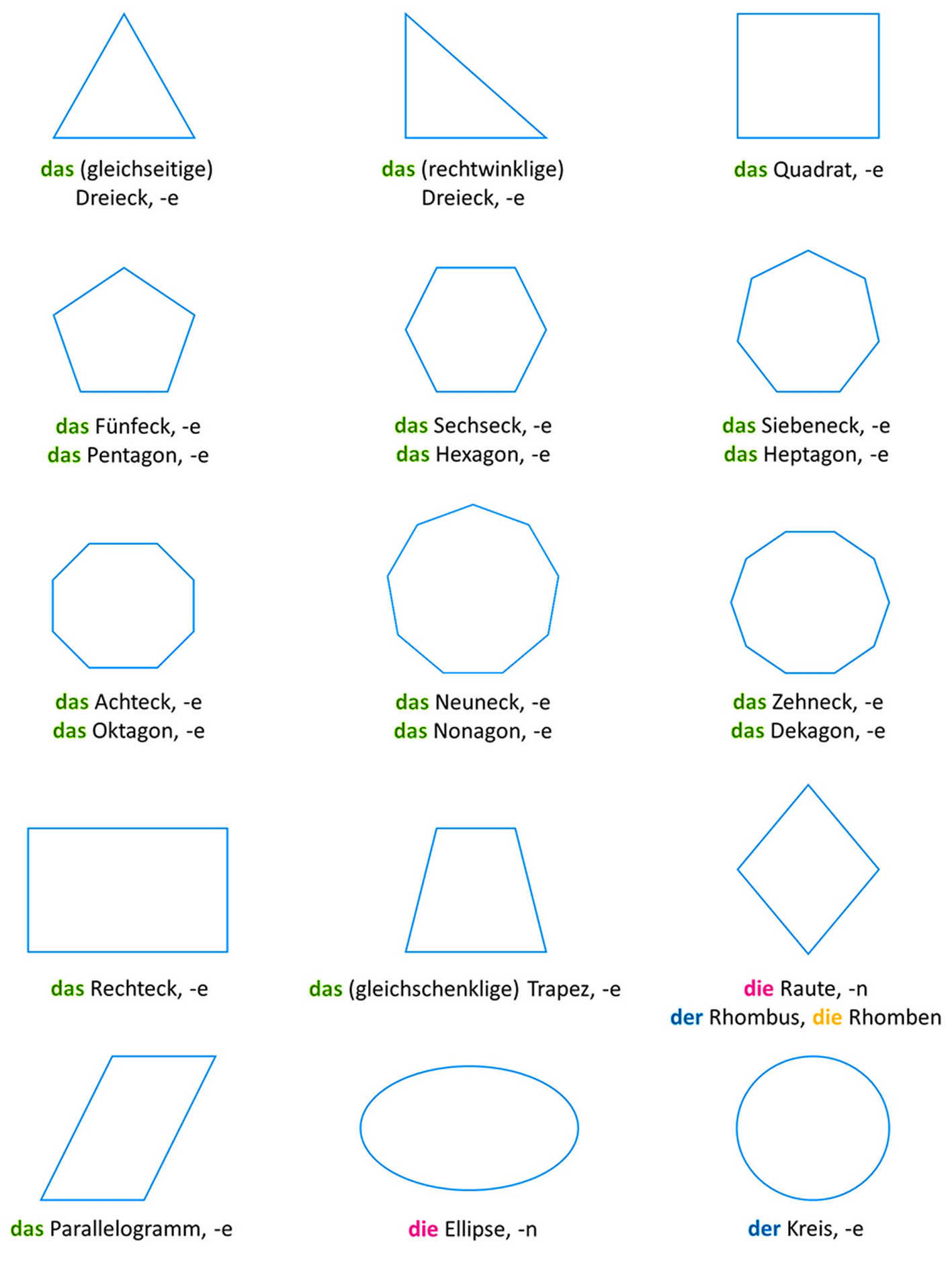Benutzer: Gast

Wörterbuch (en): Parallelogram / Rhomboid

11. Parallelogram / Rhomboid
2. Triangle
3. Square
4. Pentagon
5. Hexagon
6. Heptagon
7. Octagon
8. Nonagon
9. Decagon
10. Rectangle
11. Trapeze
12. Diamond / Rhombus
13. Ellipse
14. Circle
de: Parallelogramm (nt) / Rhomboid (nt)
fr: Parallélogramme (m)
it: Parallelogramma (f)
es: Paralelogramo (m)

A convex flat quadrilateral where opposite sides are parallel. Parallelograms are special trapezoids and two-dimensional parallelepipeds. Rectangle, diamond and square are special cases of the parallelogram.